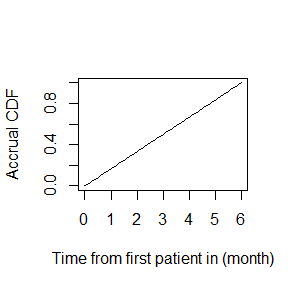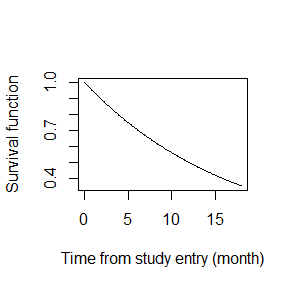# Basic functionalities

#### 2019-12-15

This vignette walks through the basic functions in npsurvSS. By the end, users should be able to:

• create an object that captures the distributional assumptions for a treatment arm
• visualize the distributional assumptions
• calculate power and sample size for a two-arm study
• approximate an event-driven study by a time-driven study
• simulate a two-arm study

## Creating objects of class “arm”

The cornerstone of npsurvSS lies in objects of class “arm”. These objects are lists that capture for a treatment arm assumptions regarding its sample size, accrual, survival, censoring, and duration of follow-up. Once created, they serve as inputs for other functions, including functions for power/sample size calculation and trial simulation.

The following code creates two arms, an active arm and a control arm. Both arms will accrue 120 patients uniformly over 6 months and follow them for an additional 12 months. Patients will be subjected to loss of follow-up at an exponential rate of 0.00578. active and control patients will experience event at exponential rates of 0.0462 and 0.0578, respectively. The hazard ratio between the two arms is therefore approximately 0.8.

library(npsurvSS)
active <- create_arm(size=120,
accr_time=6,
surv_scale=0.0462,
loss_scale=0.00578,
follow_time=12)
control <- create_arm(size=120,
accr_time=6,
surv_scale=0.0578,
loss_scale=0.00578,
follow_time=12)

In practice, investigators seldom consider exponential distributions on the hazard rate scale. Instead, they consider the median survival or survival probability at some milestone t. We have defined additional functions to facilitate this practice. per2haz is a simple code that can convert an exponential survival percentile to the hazard rate and vice versa.

active <- create_arm(size=120,
accr_time=6,
surv_scale=per2haz(15), # corresponds to 15 month median
loss_scale=per2haz(120), # corresponds to 120 month median
follow_time=12)
control <- create_arm(size=120,
accr_time=6,
surv_scale=per2haz(12), # corresponds to 12 month median
loss_scale=per2haz(120),
follow_time=12)
per2haz(15) # convert median survival to hazard rate
#>  0.04620981
per2haz(0.0462) # convert hazard rate to median survival
#>  15.00319

Alternatively, create_arm_lachin allows investigators to specify exponential survival and censoring distributions by providing median survivals or milestone survivals.

active <- create_arm_lachin(size=120,
accr_time=6,
surv_median=15,
loss_milestone=c(120, 0.5), # corresponds to 120 month median
follow_time=12)
control <- create_arm_lachin(size=120,
accr_time=6,
surv_milestone=c(12, 0.5), # corresponds to 12 month median
loss_median=120,
follow_time=12)
class(active)
#>  "list"   "lachin" "arm"

Note that objects created by create_arm_lachin belong to class “lachin” and “arm”. “lachin” is a subclass of “arm”. It is named after the class of distributions considered by Lachin (1986), which covers uniform/truncated-exponential accrual, exponential survival, and exponential censoring. Check out the R Documentation ?create_arm for examples of “arm” objects with more sophisticated assumptions, such as piecewise-uniform accrual, piecewise-exponential/Weibull survival, and Weibull censoring. While objects created by create_arm_lachin are always of class “lachin” and “arm”, objects created by create_arm are always of class “arm”, but not necessarily of class “lachin”.

## Visualizing distributional assumptions

Having created an “arm” object, visualizing its assumptions is easy. For example, the following code plots the accrual cumulative distribution function (CDF):

x <- seq(0, 6, 0.1)
plot(x, paccr(q=x, arm=control),
xlab="Time from first patient in (month)",
ylab="Accrual CDF",
type="l")Likewise, the survival function:

x <- seq(0, 18, 0.1)
plot(x, psurv(q=x, arm=control, lower.tail=F),
xlab="Time from study entry (month)",
ylab="Survival function",
type="l")Just as pbinom in the R package stats is accompanied by functions for the density, quantile, and random generation, paccr is similarly accompanied by daccr, qaccr, and raccr. Distribution functions psurv and ploss are further accompanied by hsurv and hloss for the hazard.

## Calculating power and sample size

Given an active arm and a control arm, calculating power and sample size is also easy. The following code calculates power under the default setting of an unweighted log-rank test with one-sided alpha 0.025:

power_two_arm(control, active)
#>  0.2366524

To calculate power for other tests:

# unweighted log-rank
power_two_arm(control, active, test=list(test="weighted logrank"))
#>  0.2366524
# Gehan-Breslow weighted log-rank
power_two_arm(control, active, test=list(test="weighted logrank", weight="n"))
#>  0.2210357
# difference in 12 month survival
power_two_arm(control, active, test=list(test="survival difference", milestone=12))
#>  0.2050328
# ratio of 12 month RMST
power_two_arm(control, active, test=list(test="rmst ratio", milestone=12))
#>  0.1823979

Power for multiple tests can be calculated simulateously:

power_two_arm(control, active, test=list(list(test="weighted logrank"),
list(test="weighted logrank", weight="n"),
list(test="survival difference", milestone=12),
list(test="rmst ratio", milestone=12)
))
#>   test     power
#> 1    1 0.2366524
#> 2    2 0.2210357
#> 3    3 0.2050328
#> 4    4 0.1823979

To calculate sample size required to achieve 80% power:

size_two_arm(control, active,
test=list(list(test="weighted logrank"),
list(test="weighted logrank", weight="n"),
list(test="survival difference", milestone=12),
list(test="rmst ratio", milestone=12)
))
#>   test       n0       n1        n       d0       d1        d
#> 1    1 609.7478 609.7478 1219.496 339.3015 292.4822 631.7837
#> 2    2 663.7018 663.7018 1327.404 369.3249 318.3627 687.6876
#> 3    3 729.6092 729.6092 1459.218 405.9998 349.9770 755.9768
#> 4    4 848.3117 848.3117 1696.623 472.0532 406.9159 878.9692

Note that size_two_arm returns the required sample size n and expected number of events d (per arm and total). When calculating the required sample size per arm, it considers as input the specified ratio between the two arms (e.g. 120:120) while ignoring their individual values (e.g. 120 and 120). Thus, the following two “arm” objects result in the same sample size calculation for the unweighted log-rank test:

control_new <- control
active_new  <- active
control_new$size <- 1 active_new$size   <- 1
size_two_arm(control_new, active_new)
#>        n0        n1         n        d0        d1         d
#>  609.7478  609.7478 1219.4956  339.3015  292.4822  631.7837

Sample size for a trial with 2:1 randomization in favor of the active arm can be calculated like so:

active_new$size <- 2 size_two_arm(control_new, active_new) #> n0 n1 n d0 d1 d #> 445.9943 891.9886 1337.9830 248.1789 427.8668 676.0457 ## Event-driven trials By containing the keys follow_time and total_time, “arm” objects intrinsically apply to time-driven trials that end when a fixed period of time has elapsed after the last patient in. However, they can also be used to approximate event-driven trials, trials in which the study ends when a desired number of events has been observed. Specifically, a trial requiring d events can be approximated by a trial of length t, where the expected number of events at t is equal to d. The functions exp_events and exp_duration can be useful for this purpose: exp_events(control, active) # expected number of events #>  124.3367 tau <- exp_duration(control, active, d=150) # study duration for expected number of events to equal d tau #>  23.75 Therefore, under the given assumptions, a trial requiring 150 events can be approximated by a 23.75-month long trial. When updating the trial duration in an “arm” object, it is important to update both the follow_time and total_time to ensure their consistency: control_new <- control control_new$total_time  <- tau
control_new$follow_time <- tau - control_new$accr_time
active_new  <- active
active_new$total_time <- tau active_new$follow_time  <- tau - active_new$accr_time exp_events(control_new, active_new) # check expected number of events #>  149.975 ## Simulating a trial Finally, time-driven and event-driven trials can be simulated using the following code: trial1 <- simulate_trial(control, active, duration=18) head(trial1, 5) #> arm time.accr time.obs time.total censor reason #> 81 0 0.137422475 0.9393870 1.076810 1 event #> 25 0 1.471682324 0.0696626 1.541345 1 event #> 144 1 0.003143816 1.6624453 1.665589 1 event #> 107 0 0.454901079 1.2542002 1.709101 1 event #> 186 1 1.043860969 1.0382043 2.082065 1 event table(trial1$arm, trial1$reason) #> #> administration event followup #> 0 52 63 5 #> 1 62 53 5 max(trial1$time.total)
#>  18

trial2 <- simulate_trial(control, active, events=150)
head(trial2, 5)
#>     arm time.accr  time.obs time.total censor reason
#> 213   1 0.4687554 0.6582531   1.127008      1  event
#> 40    0 0.4848245 0.6663861   1.151211      1  event
#> 44    0 0.7151992 0.4879829   1.203182      1  event
#> 106   0 0.5887742 1.3522716   1.941046      1  event
#> 9     0 0.7832331 1.3743750   2.157608      1  event
sum(trial2$censor) #>  150 If both duration and events are provided, the study will end whenever one of the criteria is met: trial3 <- simulate_trial(control, active, duration=18, events=150) max(trial3$time.total)
#>  18
sum(trial3$censor) #>  128 Should it be desired, investigators may also simulate complete data (accrual, survival, censoring) for each individual treatment arm. Note that no cutoff (by number of events or time) is applied. Hence, no patients are administratively censored: control_sim <- simulate_arm(control) head(control_sim, 5) #> arm time.accr time.obs time.total censor reason time.surv time.loss #> 1 1 5.3056575 11.756965 17.062622 1 event 11.756965 234.1555 #> 2 1 2.4190124 24.820609 27.239621 1 event 24.820609 368.0955 #> 3 1 2.5310937 5.937343 8.468437 1 event 5.937343 119.1653 #> 4 1 0.5877304 5.025779 5.613509 1 event 5.025779 383.5989 #> 5 1 1.0893293 17.136234 18.225563 1 event 17.136234 196.1827 table(control_sim$arm, control_sim\$reason)
#>
#>     event followup
#>   1   108       12